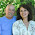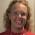Two algebra 1 word problems (systems of linear equations)

Here are two problems for you to solve... OR to learn from me when I solve them. Both problems are for algebra 1, and use a system of 2 linear equations.

By the way, the comments have some wonderful ideas for solving these mentally, without using algebra. So please read them too!

Problem: John bought red pens for \$4 apiece and blue pens for \$2.80 apiece. If John purchased a total of 24 pens for \$84, how many red pens did he purchase?

Solution: This is a typical problem that will have two variables and two equations.

Let r be the amount of red pens he buys, and b be the amount of blue pens he buys.

We get our first equation from this sentence: "He bought a total of 24 pens." So, r + b = 24.

We get the second equation from the fact that his total purchases were worth \$84, and red pens cost \$4, and blue pens cost \$2.80

4r + 2.8b = 84

Now, just solve this system of two equations using your preferred method.

r + b = 24
4r + 2.8b = 84

I will multiply the top equation by -4, then add the two equations.

-4r - 4b = -96
4r + 2.8b = 84
-------------------
-1.2b = -12
From this, b = 10.

Then since r + b = 24, r must be 14.

Problem: In a group of 60 workers, the average salary is \$80 a day per worker.  If some of the workers earn \$75 a day and all the rest earn \$100 a day, how many workers earn \$75 a day?

Solution: To get started, first find out what are the unknowns. In this case, there are two. Some workers earn \$75 a day, and some earn \$100 (two quantities).

Let A = workers who earn \$75 a day.
Let B = workers who earn \$100 a day.

We need to have both variables when building the equations, even though the problem only asks for A.

THEN we need to somehow use the information given to build two equations. That is because to solve for two unknowns, you need two equations.

Well, there are 60 workers, so our first equation is pretty easy: A + B = 60.

Now, the first sentence may throw you off... but actually, the concept of average is not coming into the picture very much. The info in the first sentence is ONLY used to find out one useful fact: that the group of workers earns 60 x \$80 = \$4,800 in TOTAL each day. We use that to build our second equation, which has to do with the total earnings:

75A + 100B = 4,800

Now we have our two linear equations, and all that remains is to solve the system using any standard technique.

A + B = 60
75A + 100B = 4,800

Multiply the top equation by -100, and then add the two equations together:

-100A - 100B = -6000
75A + 100B = 4,800
-----------------------------
-25A = -1,200
A = 48

Then, B must be 12.

Check: 48 x \$75 + 12 x \$100 = \$4,800. It checks.Pat B said…
Maria,
A thousand years ago when I was in alg I, I solved problems like this with the method of false position (I read about it in a puzzle book that focused on History topics)..If you bought all 24 pens in Red it would cost \$96, which is too much... in fact it is \$12 too much. Each blue pen saves \$1.20, so I will just try ten blues and the rest (14) red.. checking, that should be \$4*14=\$56 for the red, \$28.00 for the ten blue, and that comes out to \$84. I found that most text book problems could be done that way.Denise said…
Averages can also be easy to balance without algebra, especially when the numbers are so mental-math-friendly as the salary problem. To get an average of \$80: Each \$100/day man gets \$20 above average, so he must be balanced by four \$75/day men. This set of 5 men will be repeated enough times to make your total number of workers.

After a few years of teaching Singapore math, I've come to enjoy the challenge of turning algebra problems into elementary mental math. Now that my daughter is about ready to study real algebra, I hope I can switch mental gears!Rebecca Zook said…
Maria, I just wanted to say thank you so much for including my post about Gallon Man in your latest homeschooling math newsletter! I'm flattered to be included and I look forward to reading more of your blog! :)Maria Miller said…
No problem; here's a link to that newsletter for those who want to see it.Rebecca Zook said…
Maria, in case you're interested, here's another blog post about using drawing (like the Gallon Man approach) to solve elapsed time problems. I stumbled on this with a fifth grade student and he seemed to find it much more intuitive than the subtract-and-borrow method. http://www.zooktutoring.com/a-visual-way-to-solve-elapsed-time-problems/